###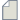Author Topic: Heisenberg exchange: QuantumATK T-2022.03  (Read 218 times)

0 Members and 1 Guest are viewing this topic.

####Dongzhe

• New ATK user
•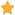• Posts: 7
• Country:• Reputation: 1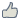##### Heisenberg exchange: QuantumATK T-2022.03
« on: April 11, 2022, 22:23 »
Dear,

For "Heisenberg exchange" calculations, why does the QuantumATK T-2022.03 perform two times self-consistent iterations (see below)?

|   0 E = -100.401 dE =  4.737280e+00 dH =  9.444844e-01                       |
|   1 E = -100.834 dE =  4.324836e-01 dH =  3.583694e-01                       |
|   2 E = -100.775 dE =  5.893032e-02 dH =  1.659173e-01                       |
|   3 E = -100.888 dE =  1.129310e-01 dH =  4.560094e-02                       |
|   4 E = -100.794 dE =  9.392506e-02 dH =  1.952596e-02                       |
|   5 E = -100.862 dE =  6.809493e-02 dH =  1.538766e-02                       |
|   6 E = -100.513 dE =  3.490458e-01 dH =  4.603800e-02                       |
|   7 E = -100.494 dE =  1.864502e-02 dH =  1.258778e-02                       |
|   8 E = -100.443 dE =  5.168407e-02 dH =  4.292440e-02                       |
|   9 E = -100.439 dE =  3.538095e-03 dH =  9.348096e-03                       |
|  10 E = -100.425 dE =  1.449278e-02 dH =  7.671024e-03                       |
|  11 E = -100.381 dE =  4.393334e-02 dH =  4.790058e-02                       |
|  12 E = -100.394 dE =  1.342525e-02 dH =  1.878510e-02                       |
|  13 E = -100.386 dE =  8.093605e-03 dH =  2.448317e-02                       |
|  14 E =  -100.37 dE =  1.619775e-02 dH =  6.779465e-03                       |
|  15 E =  -100.37 dE =  5.293426e-04 dH =  7.708884e-03                       |
|  16 E = -100.366 dE =  4.900332e-03 dH =  3.217318e-03                       |
|  17 E = -100.369 dE =  3.306735e-03 dH =  6.027327e-03                       |
|  18 E = -100.369 dE =  3.039395e-04 dH =  7.598019e-04                       |
|  19 E = -100.369 dE =  7.937560e-05 dH =  3.017771e-04                       |
|  20 E = -100.371 dE =  1.494264e-03 dH =  2.782688e-04                       |
|  21 E = -100.371 dE =  2.023463e-05 dH =  7.269279e-05                       |
|  22 E = -100.371 dE =  7.345684e-05 dH =  7.334537e-05                       |
|  23 E = -100.371 dE =  6.034397e-05 dH =  1.240043e-05                       |
|  24 E = -100.371 dE =  1.746288e-05 dH =  8.722722e-06                       |
|   0 E = -354.079 dE =  7.788081e+00 dH =  9.662794e+00                       |
|   1 E = -105.842 dE =  2.482368e+02 dH =  8.987565e+00                       |
|   2 E = -291.555 dE =  1.857125e+02 dH =  1.312920e+01                       |
|   3 E = -264.924 dE =  2.663083e+01 dH =  9.503063e+00                       |
|   4 E = -95.0854 dE =  1.698383e+02 dH =  8.783043e+00                       |
|   5 E = -113.512 dE =  1.842674e+01 dH =  2.381160e+00                       |
|   6 E =  -113.42 dE =  9.237015e-02 dH =  2.636393e+00                       |
|   7 E = -110.732 dE =  2.688205e+00 dH =  2.519853e+00                       |
|   8 E = -104.458 dE =  6.273797e+00 dH =  1.519096e+00                       |
|   9 E = -101.192 dE =  3.265449e+00 dH =  7.110295e-01                       |
|  10 E = -102.287 dE =  1.094407e+00 dH =  4.919104e-01                       |
|  11 E = -102.427 dE =  1.405807e-01 dH =  5.630493e-01                       |
|  12 E = -100.686 dE =  1.741583e+00 dH =  4.994816e-01                       |
|  13 E = -100.764 dE =  7.791032e-02 dH =  3.522554e-01                       |
|  14 E = -99.5672 dE =  1.196500e+00 dH =  4.429658e-01                       |
|  15 E = -99.6605 dE =  9.332819e-02 dH =  1.111574e-01                       |
|  16 E = -101.171 dE =  1.510161e+00 dH =  1.449661e-01                       |
|  17 E = -101.159 dE =  1.207932e-02 dH =  5.939943e-01                       |
|  18 E = -101.159 dE =  1.303078e-04 dH =  5.792142e-01                       |
|  19 E =  -101.16 dE =  1.279220e-03 dH =  5.783336e-01                       |
|  20 E =  -100.37 dE =  7.899985e-01 dH =  5.725896e-01                       |
|  21 E = -100.336 dE =  3.370613e-02 dH =  1.311135e-01                       |
|  22 E = -99.5191 dE =  8.171268e-01 dH =  1.336044e-01                       |
|  23 E = -99.8314 dE =  3.122512e-01 dH =  1.117583e-01                       |
|  24 E = -99.9239 dE =  9.249740e-02 dH =  6.342496e-02                       |
|  25 E = -99.8953 dE =  2.863251e-02 dH =  1.762643e-02                       |
|  26 E = -99.8318 dE =  6.348924e-02 dH =  1.213628e-02                       |
|  27 E = -99.8548 dE =  2.300784e-02 dH =  1.129901e-02                       |
|  28 E = -99.9246 dE =  6.980356e-02 dH =  6.431718e-03                       |
|  29 E = -99.9313 dE =  6.673782e-03 dH =  3.452789e-03                       |
|  30 E = -99.9225 dE =  8.761663e-03 dH =  6.301076e-04                       |
|  31 E = -99.9249 dE =  2.454435e-03 dH =  1.537675e-03                       |
|  32 E = -99.9253 dE =  4.006691e-04 dH =  1.465688e-03                       |
|  33 E = -99.9255 dE =  1.193658e-04 dH =  6.954636e-05                       |
|  34 E = -99.9255 dE =  2.101761e-05 dH =  6.262145e-05                       |
|  35 E = -99.9254 dE =  7.186715e-05 dH =  8.748979e-05                       |
|  36 E = -99.9255 dE =  9.048039e-05 dH =  1.382710e-04                       |
|  37 E = -99.9255 dE =  2.276349e-05 dH =  2.726686e-05                       |

Is it a bug?
Best regards,
Dongzhe Li

####maj7293

• New ATK user
•• Posts: 3
• Country: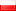• Reputation: 0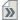##### Re: Heisenberg exchange: QuantumATK T-2022.03
« Reply #1 on: April 12, 2022, 19:04 »
Dear Dongzhe,
It seems that you've used two separate calculators in your script generator. Could you put your input to check?

####Dongzhe

• New ATK user
•• Posts: 7
• Country:• Reputation: 1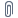##### Re: Heisenberg exchange: QuantumATK T-2022.03
« Reply #2 on: April 14, 2022, 20:15 »
Hi,
I attached my input/output. It seems a bug in the new version.
Best regards,
Dongzhe

####maj7293

• New ATK user
•• Posts: 3
• Country:• Reputation: 0##### Re: Heisenberg exchange: QuantumATK T-2022.03
« Reply #3 on: April 15, 2022, 12:45 »
I checked with the older version and it is OK there. As you guessed it probably is a bug from the newer version.
I attached the output file.####Troels-Markussen

• QuantumATK Staff
• Regular ATK user
•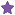• Posts: 37
• Country:• Reputation: 2
• QuantumATK staff##### Re: Heisenberg exchange: QuantumATK T-2022.03
« Reply #4 on: June 7, 2022, 14:28 »
Hi Dongzhe,

This is partly intended behavior since in our implementation, the calculation of the J_ij matrix elements require that all the atoms are located within a single unit cell. Since your configuration have some negative fractional coordinates, it will internally wrap the configuration and trigger a restart. Note, however, that the second update uses the already updated configuration and only takes 5 steps to converge (vs. 28 for the first update).

Also, I just found out now, that the check for doing a restart is too tight meaning that the program will often perform a restart even if it isn't really necessary. But again, this is just a few additional SCF steps.

Regards,
Troels